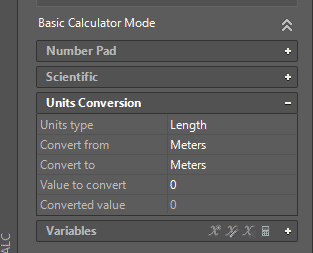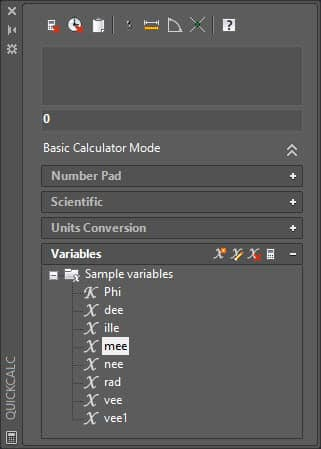With QuickCalc AutoCAD Calculator command you can measure the length of geometry, its angle and coordinate points like midpoint or centroid. You can perform complex calculations using the scientific calculator and It can also be used to convert units of length, angle, area, and volume.

To start QickCalc calculator (I will call it calculator from now on for sake of simplicity) Right click in the drawing area and select QuickCalc from the contextual menu, you can also use its command equivalent QUICKCALC. This calculator can be launched from contextual menu even if you are in the middle of a command.

In this article, I will explain how you can leverage the features of QuickCalc calculator to make your drawing and calculation task faster and efficient.

I have also prepared this video which explains this tip in detail, but if you prefer the article you can read it by scrolling down.

## Simple Calculator:

The most obvious use of a calculator tool is to perform calculations and this tool is no exception. When you launch Calculator tool a basic palette will appear with all the buttons of a calculator, you can also expand the calculator palette if it is collapsed as shown in the image below.On calculator palette, you will find a familiar calculator interface with all related buttons at your disposal. You can use the mouse to enter values in the calculator or you can use Numpad of the keyboard as well.

## Scientific Calculator:

Just below the Number Pad tab on calculator palette you will find a Scientific tab, expand it by clicking on the + icon on it. This will open a Scientific calculator for you where you can perform more complex calculations involving sines, cosines or logarithmic values.

## Unit conversion:

To convert units expand Units Conversion tab on calculator palette. Select type of unit which you want to convert from Units type field then select unit which you want to convert and in next field enter the unit in which you want it to be converted.Enter the value in Value to convert field and press tab key, the result will appear in the Converted value field.

## Length and Angle:

On the top of QuickCalc window, you will find a bar containing small icons that can be used to extract geometrical information from the drawing like distance, angle, and coordinates. Let’s start with finding the distance between two points.

To check the distance between two points click on next icon which is like a ruler with a dimension line above it. The calculator palette will disappear and now you can select two points. On selecting both the points you will see that calculator palette will appear again with the distance between both points in the result field.In a similar way as above angle of a line can also be found with respect to the positive side of the X-axis. Select the next tool as shown in the image above to measure the angle of a line with respect to the positive direction of the X-axis.

The calculator will again disappear, specify the base point for the line followed by the second reference point on the line. The angle will appear in the results field. In the image below point 1 was taken as the base point and point 2 was the second reference point on the black line.## Coordinates:

To obtain coordinates of a point click on the get coordinates icon as shown in the image below, calculator palette will temporarily disappear and your cursor will change into a point selection cursor. Click at a point in the drawing area, calculator palette will appear again with values of coordinates of the selected point in the result field.To determine coordinates of the intersection point of two lines select the next tool which looks like X letter. Again calculator window will disappear and you are prompted to select the first two points, select the points on the first line then second points on the second line. The resultant intersection point will appear in the result field of calculator palette.

## Functions or variables:

Using functions you can find many geometric parameters like midpoint and centroid. There are some predefined functions in the calculator tool but you can create your own custom function as well. List of functions can be accessed from Variables tab which is at the end of the calculator palette as shown in the image below.As an example, you can find the midpoint of a line using mee function. To use it double click on mee from sample variables tree, you will notice that (cur+cur)/2 will appear on result field of the calculator, you can type this function as well in the result field. Press enter and then click on the endpoints of a line. The coordinates of midpoint will appear in the result field of calculator palette.

## Using ‘CAL command:

You can also perform calculations directly on the command line without opening the calculator. As an example, if you want to make a circle in such a way that its center lies at a point midway between two existing points and radius is half of the radius of an existing circle then follow these steps.

Start the circle command then type ‘CAL on command prompt (don’t forget to include the Apostrophe) and press enter. Then type (END+END)/2 and press enter again, you will notice that cursor will change into a large pick box. Click at points close to the end point of the line, the center of the circle will snap to the midpoint of the line.

Now again type ‘CAL and press enter, then type Rad/2 and press enter, Click on the existing circle whose radius you want to take for reference. The required circle will be made with the radius equal to half of the radius of circle selected.

If you have questions related to this tip then let me know in the comments below.